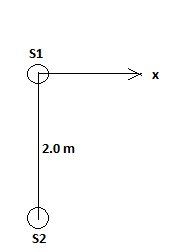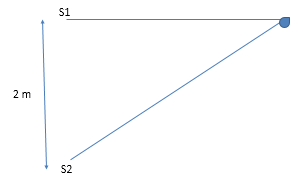# Two identical radio sources S1 and S2 separated by a distance of 2.00 m, as drawn below, emit...

## Question:

Two identical radio sources S1 and S2 separated by a distance of 2.00 m, as drawn below, emit radio waves of wavelength 0.500 m. Find the greatest distance from S1 along the x axis for which the constructive interference occurs.## Interference Of Light Wave:

According to the phenomenon of interference of light when two light waves of different amplitude superimpose each other to form the resultant wave of greater amplitude or lower amplitude depending upon the type of interference then it is known as interference of the wave.

Here the diagram shows the interference of two light waves coming from the two sources:Path difference for the constructive interference:

{eq}xS_2-xS_1=m\lambda \\ xS_2-xS1=0.5 {/eq}

From the Pythagoras theorem:

{eq}\sqrt{L^2+2^2}-L=0.5\\ \sqrt{L^2+4}-L=0.5\\ L=3.75\ m {/eq}

Thus, the greatest distance from {eq}S_1 {/eq} along the x-axis is 3.75 m.